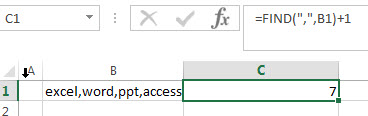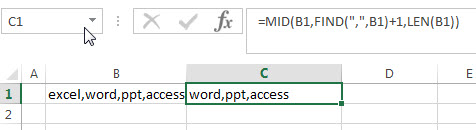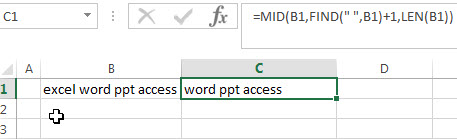# How to extract text after first comma or space

In the previous post, we talked that how to extract substring before the first comma or space or others specific characters in excel. And this post will guide you how to extract text after the first comma or space character in a text string.

## Extract text after first comma or space

If you want to get substring after the first comma character from a text string in Cell B1, then you can create a formula based on the MID function and FIND function or SEARCH function as follows:

`=MID(B1,FIND(",",B1)+1,LEN(B1))`

or

`=MID(B1,SEARCH(",",B1)+1,LEN(B1))`

Let’s see how this formula works:

=LEN(B1)

The LEN function returns the number of characters in a text string in Cell B1. The returned result goes into the MID function as its num_chars argument.

=FIND(“,”,B1)+1The FIND function returns the position of the first comma character in Cell B1. It returns 7. And then add 1 to get the position of the first character after comma character. The returned value goes into the MID function as its start_num argument.

=MID(B1,FIND(“,”,B1)+1,LEN(B1))So far, you got the values of the start_num and num_chars arguments from above FIND and LEN formula. And then the MID function extracts a substring based on the starting position and the number of the characters that you want to extract from a text string in Cell B1.

Last, if you want to extract a string after the first space character or others specific characters in a text string in cell B2, then you just need to change the comma character to space character in the above MID function, like this:

=MID(B1,FIND(” “,B1)+1,LEN(B1))### Related Formulas

• Extract Text between Parentheses
If you want to extract text between parentheses in a cell, then you can use the search function within the MID function to create a new excel formula…
• Extract Text between Brackets
If you want to extract text between brackets in a cell, you need to create a formula based on the SEARCH function and the MID function….
• Extract Text between Commas
To extract text between commas in Cell B1, you can use the following formula based on the SUBSTITUTE function, the MID function and the REPT function…..
• Extract word that starting with a specific character
Assuming that you have a text string that contains email address in Cell B1, and if you want to extract word that begins with a specific character “@” sign, you can use a combination with the TRIM function, the LEFT function, the SUBSTITUTE function ….
• Extract text before first comma or space
If you want to extract text before the first comma or space character in cell B1, you can use a combination of the LEFT function and FIND function….

### Related Functions

• Excel MID function
The Excel MID function returns a substring from a text string at the position that you specify.The syntax of the MID function is as below:= MID (text, start_num, num_chars)….
• Excel FIND function
The Excel FIND function returns the position of the first text string (sub string) within another text string.The syntax of the FIND function is as below:= FIND(find_text, within_text,[start_num])…
• Excel SEARCH function
The Excel SEARCH function returns the number of the starting location of a substring in a text string.The syntax of the SEARCH function is as below:= SEARCH  (find_text, within_text,[start_num])…
• Excel LEN function
The Excel LEN function returns the length of a text string (the number of characters in a text string).The LEN function is a build-in function in Microsoft Excel and it is categorized as a Text Function.The syntax of the LEN function is as below:= LEN(text)…
Related Posts

If Cell Contains Certain Text OR Equals Certain Text

IF cell equals certain text IF function is frequently used in Excel worksheet to return “true value” or “false value” based on the logical test result. If you want to test values to see if they equal certain text like ...

How to Extract Text between Two Text Strings in Excel

This post will guide you how to extract text between two given text strings in Excel. How do I get text string between two words with a formula in Excel. Extract Text between Two Text Strings Assuming that you have ...

How to Return a Value If a Cell Contains a Specific Text in Excel

This post will guide you how to return a value if a cell contains a certain number or text string in Excel. How do I check if a Cell contains specific text and then return another specific text in another ...

Insert The File Path and Filename into Cell

This post will guide you how to insert the file path and filename into a cell in Excel. Or how to add a file path only in a specified cell with a formula in Excel. Or how to add the ...

Sort Cells by Specific word or words

This post will guide you how to sort cells or text values in a column based on a specific word even if the word is in the text string in the cell. How do I sort cells in a column ...

Highlight Rows

This post will teach you how to highlight rows in a table with conditional formatting in Excel. You will learn that how to change the color of the entire rows if the value of cells in a specified column meets ...

How to replace all characters after the first specific character

This post will guide you how to replace all characters after the first match of a specific character with a new text string in excel. How to replace all substrings after the first occurrence of the comma character with another ...

How to extract text after the second or nth specific character (space or comma)

In the previous post, we talked that how to extract text after the first occurrence of the comma character in excel. And this post explains that how to get a substring after the second or nth occurrence of the comma ...

How to extract text before the second or nth specific character (space or comma)

Before we talked that how to extract text before the first space or comma character in excel. And this post will guide you how to extract a substring before the second or nth specific character, such as: space or comma ...

Check if Cell Contains Certain Values but do not Contain Others Values

In the previous post, we only talked that how to check a cell if contains one of several values from a range in excel. And this post explains that how to check a cell if it contains certain values or ...

Sidebar